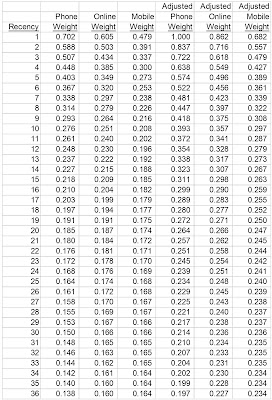## November 08, 2016

### Helping Out The Co-Ops

Our topic today is catalog marketing.

Over the past three years, performance has declined by +/- 20% for many ... some have seen increases ... but the overall trend is not good.

But catalogers can help change the dynamic.

Yes ... you!

You wanted actionable findings? Then try this.

Let's say you mail a 96 page catalog that costs \$0.55. You have a co-op segment that you like to mail. Three years ago, here's what performance looked like.
• Response Rate = 1.2%.
• Average Order Value = \$120.
• Demand per Book = 0.012 * 120 = \$1.44.
• Profit Factor = 40%.
• Cost Per Book = \$0.55.
• Profit = \$1.44 * 0.40 - \$0.55 = \$0.03.
• Profit per Order = 0.03 / 0.012 = \$2.50.
You were happy with that.

Today ... not so much. Response is down 20%. Here's what the metrics look like:
• Response Rate = 0.96%.
• Average Order Value = \$120.
• Demand per Book = 0.0096 * 120 = \$1.15.
• Profit Factor = 40%.
• Cost Per Book = \$0.55.
• Profit = \$1.15 * 0.40 - \$0.55 = (\$0.09).
• Profit per Order = (0.09) / 0.0096 = (\$9.38).
Three years ago, we were generating \$2.50 profit per order. Today, we are losing \$9.38 per order.

The co-ops are not going to be able to fix this dynamic. Only you can fix this dynamic (short-term). So get busy fixing it!!

Step 1 = Smaller Catalog. Go from 96 pages down to 64, and filter out low-performing items. On average, you'll achieve 81% of the demand on 67% of the pages. Here's what the profit and loss statement looks like.
• Response Rate = 0.78%.
• Average Order Value = \$120.
• Demand per Book = 0.0078 * 120 = \$0.94.
• Profit Factor = 40%.
• Cost Per Book = \$0.40.
• Profit = \$0.94 * 0.40 - \$0.40 = (\$0.02).
• Profit per Order = (0.02) / 0.0078 = (\$2.56).
We're closer to where we need to be.

Step 2 = 64 page Prospect Catalog - only Winning Creative and Winning Items. This will boost productivity by another 10%, +/- ... and the story changes.
• Response Rate = 0.86%.
• Average Order Value = \$120.
• Demand per Book = 0.0086 * 120 = \$1.03.
• Profit Factor = 40%.
• Cost Per Book = \$0.40.
• Profit = \$1.03 * 0.40 - \$0.40 = \$0.01.
• Profit per Order = 0.01 / 0.0086 = \$1.16.
Now we're generating profit.

When we generate profit, we can mail this segment and other segments that we could not mail otherwise. This adds new customers ... and new customers protect the future of your business.

Co-op performance is not going to improve.

Catalogers have to change.

And the changes are EASY ... how hard is it to create a prospect version of the catalog only merchandised with winning creative and winning items? This is what catalogers LOVE doing. So just do it ... help your co-op partners out and they win and you win (which means your printers win and your paper rep wins).

So go do it, right now!!